# How to Sum Numbers by Formula if Cells Are Not Equal to Certain Value in Excel

In previous article, we introduce the method to sum numbers for cells are equal to a certain. In this article, we will show you the resolution of ‘sum if cells are not equal to a certain value’ by formula with the help of Excel SUMIF function. Through demonstrate a simple instance, we will introduce you the syntax, arguments of SUMIF function, and let you know how this formula works to reach our goal. Some other functions like SUMIFS can also resolve this issue, we will also introduce SUMIFS function a little bit in the end to let you know another way of sum number with condition. After reading the article, you may have a simple understanding of SUMIF function.

EXAMPLE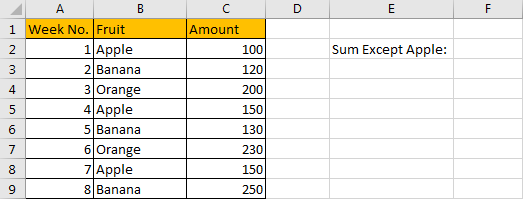Refer to above table, we can see that we sale only one kind of fruit each week. We list week number in column A, some kinds of fruits in column B, fruits are on sale alternatively. Total amount for each week for specific fruit is listed in column C.

In this instance, we want to calculate total amount for fruits except fruit ‘Apple’. That means we need to sum up numbers in column C which B column in the same row is not equal to ‘Apple’. We can apply a formula contains SUMIF function to resolve this issue.

FORMULA – SUMIF FUNCTION

Step 1: Select B2:B9, then in Name Box define a new name for this range, for example ‘Fruit’.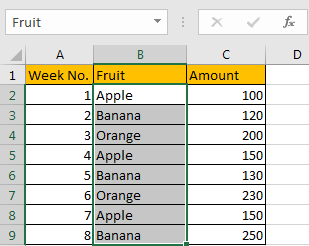Step 2: Select C2:C9, in Name Box define a new name for this range, for example ‘Amount’.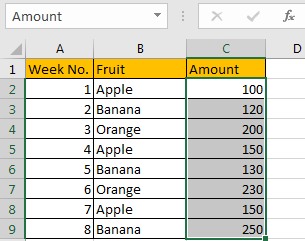Step 3: In F2, enter the formula =SUMIF(Fruit,”<>Apple”,Amount).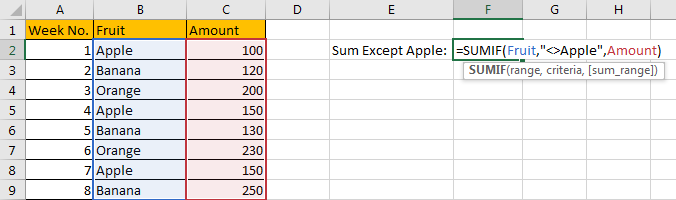Step 4: Press Enter after typing the formula.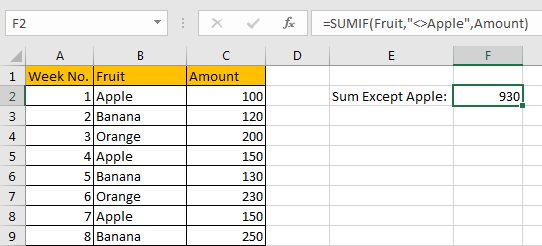We can see in column A there are five cells are not equal to text ‘Apple’, the corresponding amounts are 120 (in cell C3), 200 (in cell C4), 130 (in cell C6), 230 (in cell C7) and 250 (in cell C9), so the total is 120+200+130+230+250=930. The formula works correctly.

SUMIF FUNCTION INTRODUCTION

SUMIF function can be seen as SUM+IF, it can handle one combination of ‘criteria range’ and ‘criteria’.

For SUMIF function, the syntax is:

=SUMIF (range, criteria, [sum_range]). Argument in [] is optional. If sum range is omitted, SUMIF will sum up all numbers in range argument.

SUMIF function supports wildcards like asterisk ‘*’ and question mark ‘?’, it also supports logical operators within its arguments. If wildcards or logical operators are required, they should be enclosed into double quotes (““) with text.

The usage of wildcards:

1. An asterisk (*) means one or more characters.
2. A question mark (?) means one character.
3. The position of asterisk or question mark means the character(s) position relative to the entered part.

The usage of logical operators:

1. “>” – greater than
2. “<” – less than
3. “<>” – not equal to

ALL ARGUMENTS

SUMIF – RANGE

In our instance, B2:B9 is the ‘range’ obviously. We define this range with name ‘Fruit’ in precondition.

In the formula bar, select ‘Fruit’, press F9, values in this range are listed in an array.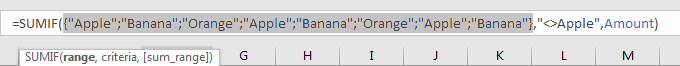SUMIF – CRITERIA

We can supply a criterion to check if values in criteria range meet this criterion. As we want to calculate total amount for fruits except ‘Apple’, equivalent to calculate total amount for both Banana and Orange. If we sum numbers based on fruit which is equal to ‘Banana’ or ‘Orange’, ‘Banana’ and ‘Orange’ cannot be both included in formula as we can only supply one criterion. So, in criteria position we enter ‘<>Apple’,’<>’ means ‘is not equal to’. We need to look up all cells which are not equal to ‘Apple’ from criteria range. SUMIF function supports text, but they should also be enclosed into double quotes “ “.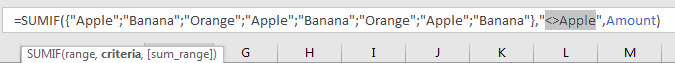SUMIFS – SUM RANGE

C2:C9 is the sum range. We define this range with name ‘Amount’ in precondition, it is easy to understand than just entering ‘C2:C9’ in sum range position in formula. In the formula bar, amounts are displayed in proper order in an array: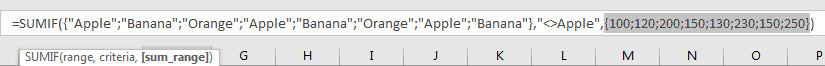HOW FORMULA WORKS

Below analysis can help us know the process of this formula reaching the goal step by step clearly.

After introducing each argument, we get two arrays and a text within double quotes, the formula is converted into below format in the formula bar.

`=SUMIF({"Apple";"Banana";"Orange";"Apple";"Banana";"Orange";"Apple";"Banana"},"<>Apple",{100;120;200;150;130;230;150;250})`

See screenshot below: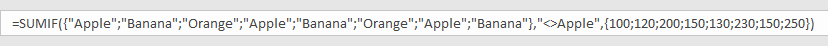As mentioned above, range and criteria are:

Criteria range: {“Apple”;”Banana”;”Orange”;”Apple”;”Banana”;”Orange”;”Apple”;”Banana”}

Criteria: “<>Apple”

Compare each text in ‘range’ array with supplied criteria ‘is not equal to Apple’. If “Apple” occurs, it doesn’t meet the supplied criteria, so mark a ‘False’ in this position; if text is not ‘Apple’, then mark a ‘True’ in this position.

So, after comparing we get below array:

{False;True;True;False;True;True;False;True}

Convert ‘True’ to ‘1’ and ‘False’ to ‘0’:

{0;1;1;0;1;1;0;1}

Now, we have below two arrays:

Array 1: {100;120;200;150;130;230;150;250} – sum range

Array 2: {0;1;1;0;1;1;0;1} – if cell <> ‘Apple’, 1 is displayed.

We list the two arrays in two rows, and create a new array ‘Array3’ to save numbers based on different values in array2. For example, in array1, value will be copied to array3 only if array2 value in the same column is “1”, otherwise, “0” will be recorded in array3 instead of the original value. So, we get below table finally: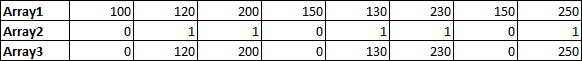In array2, 1 means in this position the original value is not equal to ‘Apple’; Array1 records amount for each kind of fruit in array2. Above all, numbers which are not zero in array3 are the amounts of ‘Banana’ and ‘Orange’ after filtering. Now, sum up all values in array3.

120+200+130+230+250=930

1. To understand the formula easily, we define range name in precondition. If you ignore this step, you can directly enter the range reference in formula.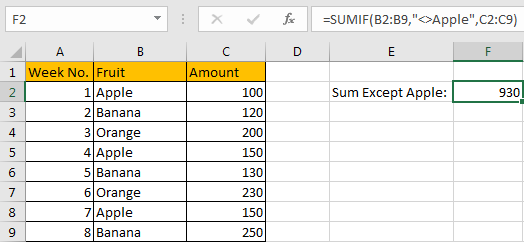2. For this instance, you can also apply SUMIFS function.

Enter =SUMIFS(Amount,Fruit,”<>Apple”) instead of applying SUMIF formula.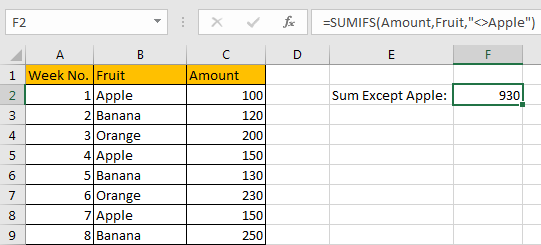We can get the same result based on above formula. You can see that the order of arguments is a little different than SUMIF function.

SUMIFS function can be seen as SUM+IFS, so it can handle multiple criteria range and criteria combinations. As we mentioned above, we want to sum numbers for ‘Banana’ and ‘Orange’, so, can we supply two criteria ‘Banana’ and ‘Orange’ within SUMIFS function in this case? Like =SUMIFS(Amount,Fruit,”Banana”,Fruit,”Orange”) ? In fact, we cannot set ‘Banana’ and ‘Orange’ as two criteria, because in criteria range there is no one fruit can meet both two criteria ‘Banana’ and ‘Orange’. Fruit is either banana or orange, it cannot be both. So, if we enter =SUMIFS(Amount,Fruit,”Banana”,Fruit,”Orange”) to sum numbers, it doesn’t work.

SUMMARY

1. SUMIF function can handle one group of criteria ranges and criteria. Sum range is optional. Sum range is the last argument among all arguments.

2. SUMIFS function can handle multiple groups of criteria ranges and criteria. Sum range is the first argument among all arguments.

3. They both support user defined range name.

4. They both support wildcard.

5. They both support logical operators.

### Related Functions

• Excel SUMIF Function
The Excel SUMIF function sum the numbers in the range of cells that meet a single criteria that you specify. The syntax of the SUMIF function is as below:=SUMIF (range, criteria, [sum_range])…
• Excel SUMIFS Function
The Excel SUMIFS function sum the numbers in the range of cells that meet a single or multiple criteria that you specify. The syntax of the SUMIFS function is as below:=SUMIFS (sum_range, criteria_range1, criteria1, [criteria_range2, criteria2], …)…